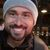Announcements
The way to achieve your own success is the willingness to help somebody else. Go for it!
cancel
Showing results for
Did you mean:Partner - Contributor III

## Set Analysis limitation, bug or misunderstanding?

Hi there! 😃

I'm trying to calculate averages without outliers. I've used the FRACTILE function to determine which numbers are my outliers and exclude them from my AVG. Basically, the Function 1 (in this example) brings a value that is not the same when it is inside a set analysis condition, on the same tuple.

[Function 1] FRACTILE({<[QTD]-={0}>} [QTD],0.75)+(1.5*(FRACTILE({<[QTD]-={0}>} [QTD],0.75)-FRACTILE({<[QTD]-={0}>} [QTD],0.25)))
RESULT: 599,75

ROUND(AVG({<[QTD]-={">=\$(=FRACTILE({<[QTD]-={0}>} [QTD],0.75)+(1.5*(FRACTILE({<[QTD]-={0}>} [QTD],0.75)-FRACTILE({<[QTD]-={0}>} [QTD],0.25))))<=-214,25"}>}[QTD]))
RESULT: 157 (should be 191)

ROUND(AVG({<[QTD]-={">=599,75<=-214,25"}>}[QTD]))
RESULT: 191

In the attached file, there is a column hardcoded for one dimension value to show this problem. Please, I appreciate any help.

OBS: Previous calculation at load script would be a solution for this scenario, but it's not factible at the real one because I have many others metrics and dimensions to do the same calc. Must be dynamic.

Thanks and regards!

Labels (4)

• ### Set Analysis

1 Solution

Accepted SolutionsPartner - Master III

HI

your expression should look like this

avg(aggr(if(QTD<= \$(LSup([QTD],[REGIONAL])) and QTD >=\$(LInf([QTD],[REGIONAL])) ,QTD),ID,REGIONAL))

you can't use set analysis because it will return one number to all regions and you want different limits to each region

4 RepliesPartner - Master III

HI

your expression should look like this

avg(aggr(if(QTD<= \$(LSup([QTD],[REGIONAL])) and QTD >=\$(LInf([QTD],[REGIONAL])) ,QTD),ID,REGIONAL))

you can't use set analysis because it will return one number to all regions and you want different limits to each regionPartner - Contributor III
Author
Hi!

It's worked! Nice! But do you know why the function below doesn't?

ROUND(AVG({<[QTD]-={">=\$(=\$(LSup([QTD],[REGIONAL]))<=\$(=\$(LInf([QTD],[REGIONAL])))"}>}[QTD]))

Thanks!Partner - Master III

because

you can't use a nested aggregation inside aggregation

the aggr convert the inner part to virtual table and than you can use an aggregation functionPartner - Contributor III
Author
Thanks, @lironbaram!Tags
Community Browser